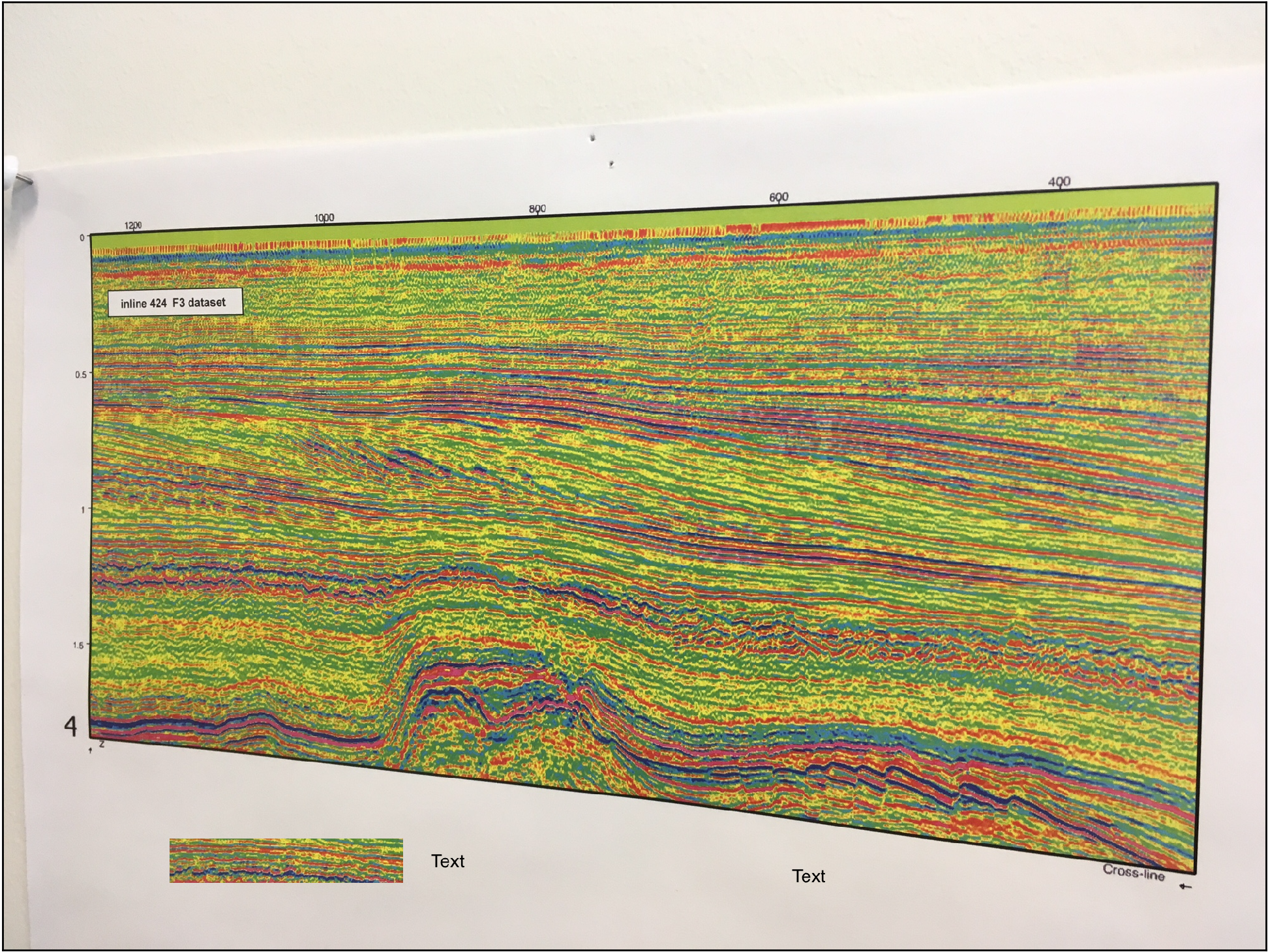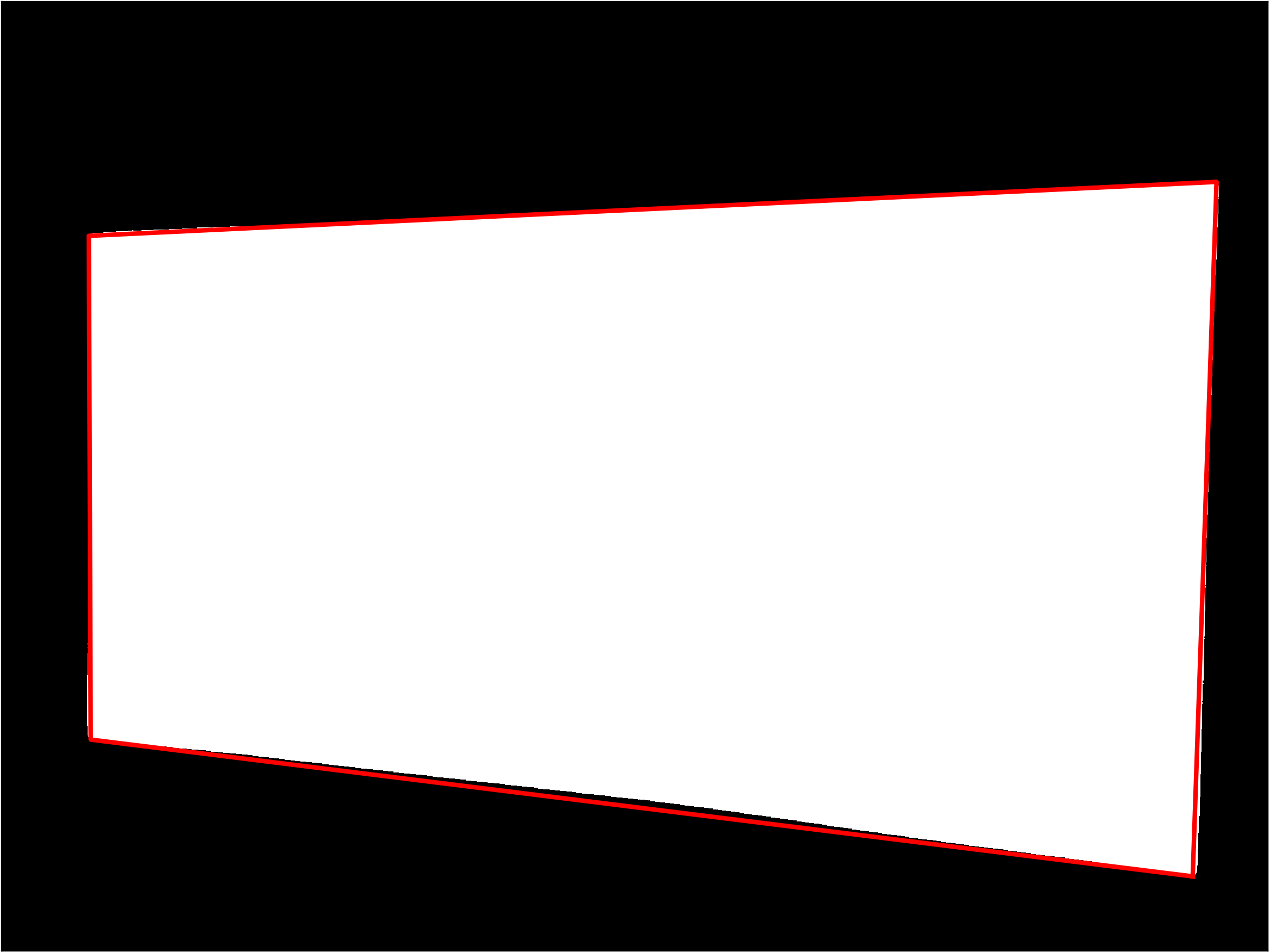# Computer vision in geoscience: recover seismic data from images – part 2

In part 1 of this short series I demonstrated how to detect the portion occupied by the seismic section in an image (Figure 1).Figure 1

The result was a single binary image with the white object representing the pixels occupied by the seismic section (Figure 2).Figure 2

You can download from GitHub all the tools for the automated workflow (including both part 1 and part 2, and some of the optional features outlined in the introduction) in the module mycarta.py, as well as an example Jupyter Notebook showing how to run it.

Next I want to use this binary object to derive a transformation function to rectify to a rectangle the seismic section in the input image.

The first step is to detect the contour of the object. Notice that because we used morphological operations it is not a perfect quadrilateral: it has rounded corners and some of the sides are bent, therefore the second step will be to approximate the contour with a polygon with enough tolerance to ensure it has 4 sides only(this took some trial and error but 25 turned out to be a good value for the parameter for a whole lot of test images I tried).

In reality, the two steps are performed together using the functions find_contours (there is only one to find, reallyand approximate_polygon from the skimage.measure module, as below:

```contour = np.squeeze(find_contours(enhanced, 0))
coords = approximate_polygon(contour, tolerance=25)```

The variable coords contains the coordinate for the corner points of the polygon (the first point is repeated last to close the polygon), which in Figure 3 I plotted superimposed to the input binary object.Figure 3 – approximated polygon

A problem with the output of  approximate_polygon is that the points are not ordered; to solve it I adapted a function from a Stack Overflow answer to sort them based on the angle from their centroid:

```def ordered(points):
x = points[:,0]
y = points[:,1]
cx = np.mean(x)
cy = np.mean(y)
a = np.arctan2(y - cy, x - cx)
order = a.ravel().argsort()
x = x[order]
y = y[order]
return np.vstack([x,y])```

I call the function as below to get the corners in the contour without the last one (repetition of the first point).

`sortedCoords = ordered(coords[:-1]).T`

I can then plot them using colors in a predefined order to convince myself the indeed are sorted:

```plt.scatter(sortedCoords[:, 1], sortedCoords[:, 0], s=60,
color=['magenta', 'cyan', 'orange', 'green'])```Figure 4 – corners sorted in counter-clockwise order

The next bit of code may seem a bit complicated but it is not. With coordinates of the corners known, and their order as well, I can calculate the largest width and height of the input seismic section, and I use them to define the size of the registered output section, which is to be of rectangular shape:

```w1 = np.sqrt(((sortedCoords[0, 1]-sortedCoords[3, 1])**2)
+((sortedCoords[0, 0]-sortedCoords[3, 0])**2))
w2 = np.sqrt(((sortedCoords[1, 1]-sortedCoords[2, 1])**2)
+((sortedCoords[1, 0]-sortedCoords[2, 0])**2))

h1 = np.sqrt(((sortedCoords[0, 1]-sortedCoords[1, 1])**2)
+((sortedCoords[0, 0]-sortedCoords[1, 0])**2))
h2 = np.sqrt(((sortedCoords[3, 1]-sortedCoords[2, 1])**2)
+((sortedCoords[3, 0]-sortedCoords[2, 0])**2))

w = max(int(w1), int(w2))
h = max(int(h1), int(h2))```

and with those I define the coordinates of the output corners used to derive the transformation function:

```dst = np.array([
[0, 0],
[h-1, 0],
[h-1, w-1],
[0, w-1]], dtype = 'float32')```

Now I have everything I need to rectify the seismic section in the input image: it is warped using homologous points (the to sets of four corners) and a transformation function.

```dst[:,[0,1]] = dst[:,[1,0]]
sortedCoords[:,[0,1]] = sortedCoords[:,[1,0]]
tform = skimage.transform.ProjectiveTransform()
tform.estimate(dst,sortedCoords)
warped =skimage.transform.warp(img, tform, output_shape=(h-1, w-1))```

Notice that I had to swap the x and y coordinates to calculate the transformation function. The result is shown in Figure 5: et voilà!Figure 5 – rectified seismic section

You can download from GitHub the code to try this yourself (both part 1 and part 2, and some of the optional features outlined in the introduction, like removing the rectangle with label inside the section) as well as an example Jupyter Notebook showing how to run it.